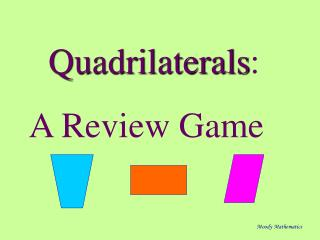Download PresentationQuadrilaterals : A Review Game

Loading in 2 Seconds...

# Quadrilaterals : A Review Game - PowerPoint PPT Presentation

Quadrilaterals : A Review Game. Moody Mathematics. Go To The Head of the Class--Review Game. Moody Mathematics. Rules : A question starts with a player. If that player answers correctly he/she keeps his/her seat. Moody Mathematics.I am the owner, or an agent authorized to act on behalf of the owner, of the copyrighted work described.
Download Presentation## Quadrilaterals : A Review Game

An Image/Link below is provided (as is) to download presentation

Download Policy: Content on the Website is provided to you AS IS for your information and personal use and may not be sold / licensed / shared on other websites without getting consent from its author.While downloading, if for some reason you are not able to download a presentation, the publisher may have deleted the file from their server.

- - - - - - - - - - - - - - - - - - - - - - - - - - E N D - - - - - - - - - - - - - - - - - - - - - - - - - -
Presentation Transcript
1. Quadrilaterals: A Review Game Moody Mathematics

2. Go To The Head of the Class--Review Game Moody Mathematics

3. Rules: A question starts with a player. If that player answers correctly he/she keeps his/her seat. Moody Mathematics

4. If the player misses, then the question goes to the next player. If missed, it goes to the next player... Moody Mathematics

5. …and continues until someone gets it correct. That student moves to the seat where the question started... Moody Mathematics

6. …and everyone who missed the question moves back one seat. Moody Mathematics

7. The object of the game is to occupy and keep the #1 seat. Moody Mathematics

8. Questions with a limited number of possible responses will not be offered indefinitely. A substitute question will be posed. Moody Mathematics

9. It is important to listen to previous responses. They will not be repeated for you. Moody Mathematics

10. Have paper and pencil, formula sheet, and calculator ready. Moody Mathematics

11. The first person to receive a question will have a maximum of 30 seconds to answer. Moody Mathematics

12. Every person after that has only 10 seconds to reply. Be ready when called upon. Moody Mathematics

13. Terms and Definitions: Moody Mathematics

14. What is the term for a quadrilateral with exactly 1 pair of opposite sides parallel? Moody Mathematics

15. Answer: Trapezoid Moody Mathematics

16. What is the term for an equilateral quadrilateral? Moody Mathematics

17. Answer: Rhombus Moody Mathematics

18. What is the term for a regular quadrilateral? Moody Mathematics

19. Answer: Square Moody Mathematics

20. What is the term for a segment that connects 2 non-consecutive vertices of a polygon? Moody Mathematics

21. Answer: Diagonal Moody Mathematics

22. What is the term for the 2 sides of a trapezoid that are parallel? Moody Mathematics

23. Answer: Bases Moody Mathematics

24. What is the term for a quadrilateral with 2 pairs of opposite sides parallel? Moody Mathematics

25. Answer: Parallelogram Moody Mathematics

26. What is the term for a quadrilateral with 4 right angles? Moody Mathematics

27. Answer: Rectangle Moody Mathematics

28. What is the term for the 2 sides of a trapezoid that are not parallel? Moody Mathematics

29. Answer: Legs Moody Mathematics

30. What is the term for the segment that joins the midpoints of the legs of a trapezoid? Moody Mathematics

31. Answer: Midsegment Moody Mathematics

32. What is the term for a quadrilateral with 2 distinct pairs of consecutive sides congruent? Moody Mathematics

33. Answer: Kite Moody Mathematics

34. What is the term for a trapezoid with congruent legs? Moody Mathematics

35. Answer: An Isosceles Trapezoid Moody Mathematics

36. What is the term for an equiangular quadrilateral? Moody Mathematics

37. Answer: Rectangle Moody Mathematics

38. SYMMETRY Moody Mathematics

39. Which special quadrilateral has the symmetry described... Moody Mathematics

40. - 4 lines - 90o rotational Moody Mathematics

41. Answer: Square Moody Mathematics

42. - No lines - 180o rotational Moody Mathematics

43. Answer: Parallelogram Moody Mathematics

44. - 2 lines (not along diagonals) - 180o rotational Moody Mathematics

45. Answer: Rectangle Moody Mathematics

46. - 2 lines (along diagonals) - 180o rotational Moody Mathematics

47. Answer: Rhombus Moody Mathematics

48. - 1 line (along a diagonal) - No Rotational Moody Mathematics

49. Answer: Kite Moody Mathematics

50. -1 line (not along a diagonal) - No rotational Moody Mathematics